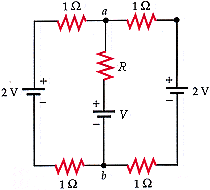# Resistance and Circuits

Hello Everyone I'm a first timer looking for some help on a Homework issue.
This thing is stumping me like crazy

## Homework Statement

For the circuit in Figure 25-63, V = 1 V and R = 4 . Find the potential difference between points a and b.V=IR

## The Attempt at a Solution

Well I calculated that the entire circuit would have a total resistance of 5 ohms. Because I calculated each border resistance in series being (1/1)+(1/1) = 1/R. Then added the middle 4. Now using the equation V=IR I solved for my current (2/5)= 0.4 A
So now to find the Potential difference I did (1-(4*0.4)) = -0.6 Volts shouldn't be negative so what in the world gives.

Last edited:

There seems to be a fundamental mistake in your calculations.
2V is not in parallel with Resistance equivalent of 5 ohms.
You have 3 voltage sources in this circuit.
You want to find the voltage between points a and b you have V=1V and R=4Ohms. so you must calculate the NET current going to R then use V=IR.

Use superposition theorem. It is the easiest way to go.

A reminder: pay attention to the direction of the currents. this is important.

Pooface, (haha love the name)

So my approach would be to find the net current going to R so would that mean finding the current from the left hand side of the circuit to a and b and just doing that twice because the other half is the same?

Yup. Dont forget there is also the third voltage source in the middle that will affect the net current.

Thats where I am getting screwed up....How does that third voltage source affect the net current? If you don't mind me asking.

Well that source is also going to give a current right? It has potential and is part of a closed loop circuit. It will not stay quiet let me assure you that.

You can see the direction of the source as well. It will be opposing the current coming in from the top.

You subtract the currents. The higher current wins and goes in that direction.

Apply superposition theorem. Use one source and short the other two. Figure out the current through R and called it I1. Then use another source and short the other two and repeat this process till you have currents accounted for each source.

Feel free to use series-parallel techniques to reduce the circuit while you are examining one source at a time.

After working on it today....saving my self to type is my answer 0.6 V?

I am getting 1.8V

Damnnit I have to redo this i must be missing something.